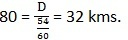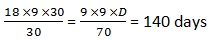## Questions On Quantitative Techniques SMQTQ040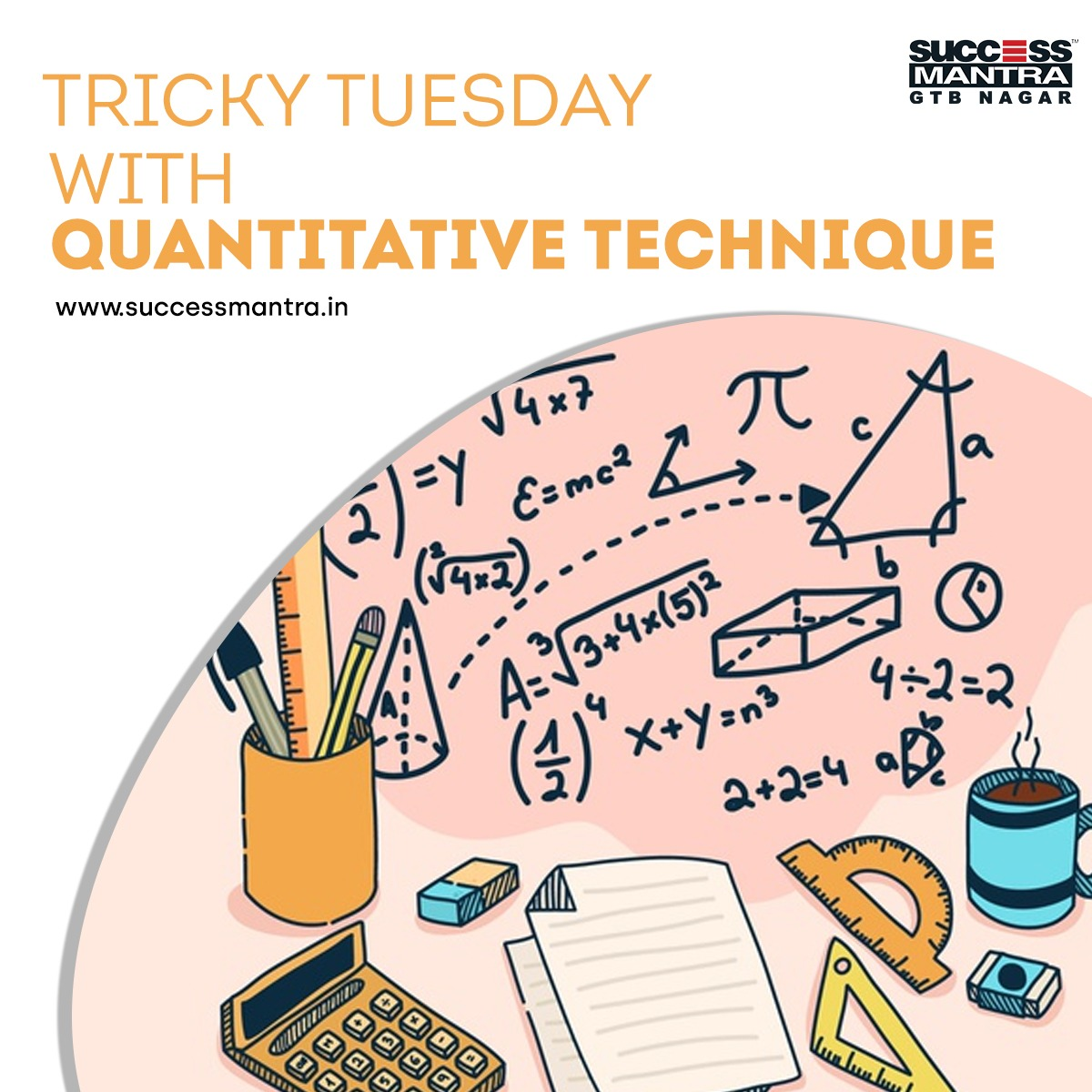1. In an organization, it is observed that every two employee share the newspaper “The Hindu” between them. Every three employee shared among them the newspaper “The Economic Times” and every four employee used to share “The Indian Express” from the library. But it is well there are only 182 newspaper came on daily basis. The number of employee in the organization:

a) 162
b) 168
c) 96
d) Cannot be determined.

2. A shopkeeper sold an article at a profit of 20%. If he had bought it at 20% less & sold it at Rs 80 less, then he earns a profit of 25%. Find the cost price of the article.

a) 350
b) 375
c) 450
d) 400

3. A rickshaw dealer buys 30 rickshaws for Rs.4725. Of these, 8 are four seaters and rests are two seaters. At what price must he sell the four seaters so that if he sells the two seaters at 3/4th of this price, he makes a profit of 40% on his outlay?

a) Rs. 180
b) Rs. 270
c) Rs. 360
d) Rs. 450

4. The difference between simple and compound interest on a certain sum of money for 2 years at 4 per cent per annum is Rs. 1. The sum of money is:

a) Rs. 600
b) Rs. 625
c) Rs. 560
d) Rs. 650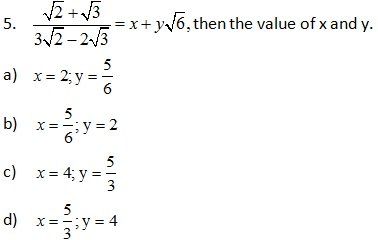a) 120
b) 123
c) 126
d) 130

7. Ram walks 40 km at 5 km/hr; he will be late by 1 hour and 20 minutes. If he walks at 8 km per hr, how early from the fixed time will he reach?

a) 1 hour and 15 minutes
b) 1 hour and 25 minutes
c) 1 hour and 40minutes
d) 1.5 hours

8. A Superfast train starts from Delhi station and reaches Agra 30 minutes late when it moves with 80 km hr and 48 minutes late when it goes 60 km hr. The distance between the two stations is:

a) 32 km
b) 38 km
c) 36 km
d) 42 km

9. 18 women work 9 hour per day to complete 30% of the work in 30 day. The rest work is done by 9 women, working 9 hour per day. Then work did in how many days.

a) 145
b) 154
c) 147
d) 140

10. Out of 11 persons, 10 persons spent Rs. 35 each for their meals. The 11th one spent Rs. 40 more than the average expenditure of all the nine. The total money spent by all of them was:

a) Rs. 492.50
b) Rs. 497.50
c) Rs. 429
d) Rs. 498.50

Explanation: Let the employee be x, then
x/2 + x/3 +x/4 = 182
On solving, X = 168

Explanation: Here C.P = 100%., S.P = 120%
If he bought at 20% less then C.P = 80%
Required S.P = 80%×125/100=100%
120% - 80 = 100%
20% = 80
C.P is 100%, Then 100% = Rs 400.

Explanation: Total investment = Rs. 4725
Total SP = 1.4 ×4725 = 6615
Now, Let the price of 4 seater be x then price of two seater will be .75x.
8x + 22 ×0.75x = 6615
24.5x= 6615 or x = 270.

Explanation: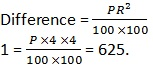Explanation: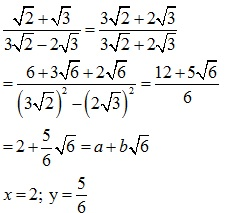Explanation: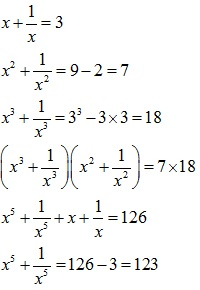Explanation: 1st case time taken = 40/5 = 8 hours
2nd case = 40/8 = 5 hours
Scheduled time = 8 hours – 80 minutes = 6 hours and 40 minutes. If Ram walks at speed of 8 km /hour, then he takes 5 hours to reach the destination and the fixed time is 6 hours and 40 minutes. He reaches early by 1 hour and 40 minutes.

Explanation: Let the right time be t. According to question
80(t + 30) = 60 (t + 48)
T = 24 minutes